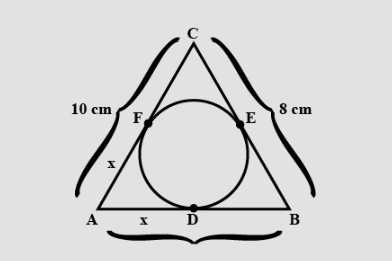Courses
Courses for Kids
Free study material
Free LIVE classes
MoreLIVE
Join Vedantu’s FREE Mastercalss

# Find the value of $AD,BF$ and $CF$ in the given figure.Verified
364.2k+ views
Hint: Use the rule of the tangents drawn from an external point to a circle subtended are equal.

We are going to use the rule that tangents drawn from an external point to a circle are equal.
Therefore,
$\Rightarrow AD = AF,BD = BE$ and $CE = CF$
Now let us take,
$\Rightarrow AD = AF = a$,
$\Rightarrow BD = BE = b$ and,
$\Rightarrow CE = CF = c$,
$AB = AD + DB = a + b = 8$ …..(1)
$BC = BE + EC = b + c = 10$ …..(2)
$AC = AF + FC = a + b = 12$ …..(3)
Add (1), (2) and (3), we get,
$2\left( {a + b + c} \right) = 30$
Taking the numbers on one side and variable on the other, we have,
$\left( {a + b + c} \right) = \frac{{30}}{2} = 15$…..(4)
Now, we are going to use (1), (2), (3) and (4), to find the value of the variables.
Subtracting (1) from (4), we get $c = 7$
Subtracting (2) from (4), we get $a = 5$
Subtracting (3) from (4), we get $b = 3$
Therefore,
$AD = a = 5cm$,
$BE = b = 3cm$
And, $CF = c = 7cm$
Note: We started by taking the rule of tangents drawn from external points to circle are equal, using this we formed equations and then solved it to get the values.
Last updated date: 29th Sep 2023
Total views: 364.2k
Views today: 5.64k